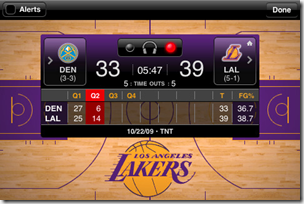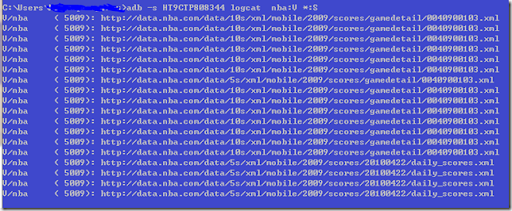# My Note on Solutions.

## Thursday, April 22, 2010

### How Nba Game Time App worksNBA game time is one of my favorite app on my nexus. It also has the Iphone Version with almost the same features.
I am wondering how does the app get the live date? is it encrypted or compressed?
it turns out the app keep polls the xml feed, here is one samplehere is one of the daily_score.xml
- <ds d="20100422" mid="1271984753522" next="http://data.nba.com/data/5s/xml/mobile/2009/scores/20100422/daily_scores.xml">
- <g i="0040900103" p="4" st="Q4" cl="10:07" r="0" ser="CLE leads series 2-0" is="1">
<b b1="TNT" b2="" b3="" />
<v t="CLE" re="2-0" s="68" see="1" />
<h t="CHI" re="0-2" s="81" see="8" />
</g>
- <g i="0040900143" p="" st="9:30 pm ET" cl="" r="0" ser="LAL leads series 2-0" is="1">
<b b1="TNT" b2="" b3="" />
<v t="LAL" re="2-0" s="0" see="1" />
<h t="OKC" re="0-2" s="0" see="8" />
</g>
- <g i="0040900163" p="" st="10:00 pm ET" cl="" r="0" ser="Series tied 1-1" is="1">
<b b1="NBA TV" b2="" b3="" />
<v t="PHX" re="1-1" s="0" see="3" />
<h t="POR" re="1-1" s="0" see="6" />
</g>
</ds>

seems I can write my app on my own by consuming the live data.

## Friday, April 16, 2010

### Some Great Android Resources